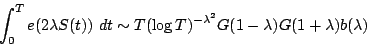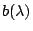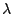# The integral of $\exp(i \lambda S(t))$

In the paper [CMP 1 794 265] by Keating and Snaith, the authors conjecture (see equation (100)) thatwheremay be expressed as an absolutely convergent product over primes and G is the Barnes double gamma function.

Ifis an integer, then the main term is 0. Ifis not an integer, then no instance of this remarkable formula has been proven (or even conjectured) before.

Back to the main index for L-functions and Random Matrix Theory.